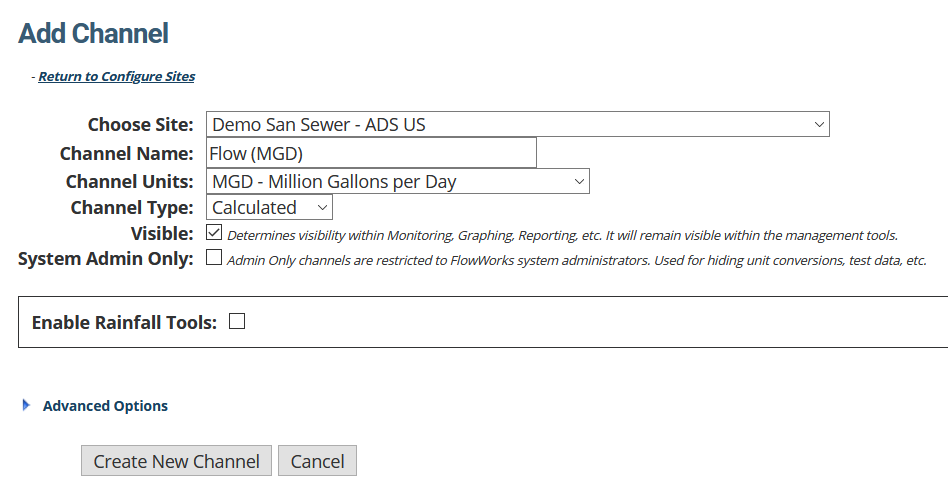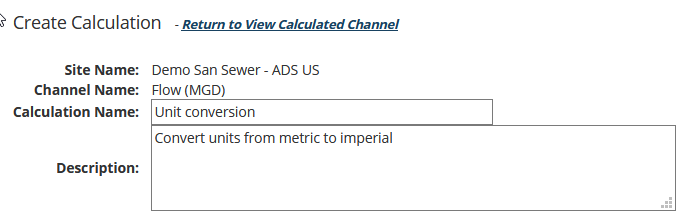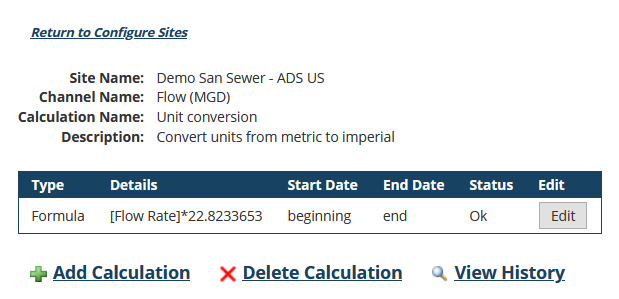# Convert Metric Data Units to Imperial

Using FlowWorks' Advanced Calculation Engine (FACE), setup a calculated channel that converts your raw Metric data to Imperial units. You can then use this calculated channel in lieu of the raw data channel in any of FlowWorks' tools.

The exampled used in this article will demonstrate how to convert volumetric flowrate units from m3/s to MGD. The method described here can be used to convert data expressed in any form of units - you will just need to define a different equation.

## 1. Open FACE

1. Click on Manage.
2. Select FACE: Configure Sites. The Configure Sites page loads.## 2. Open the Add New Channel page## 3. Create the new calculated channel

1. Expand the Choose Site menu, and select the site in which you want to store the calculated channel.
2. Type a Channel Name.
We recommend using the name of the original channel, with the units you want to use appended to the end.
For this example, we wrote "Flow (MGD)".
3. Choose the Channel Units.
For this example, we chose MGD.
4. For Channel Type, choose Calculated.
5. Ensure Visible is checked.
6. Check Enable Rainfall Tools if you want to use this channel in any of FlowWorks' rainfall tools, including I&I Analysis.
7. (Optional) Expand Advanced Options to Add This Channel to Other Sites.
The list provided only contains sites that do not already have a channel with the same name.
8. Click Create new Channel.
Your new channel saves. You are taken back to the Configure Sites page.## 4. Configure the Calculated Channel

Now that you've create the calculated channel, you need to setup the unit conversion formula.

1. Expand Choose Sites, and select the site in which the new calculated channel resides.
2. Expand Choose Calculated Channel, and select the new channel you just made.
3. Click View Calculated Channel. The View Calculated Channel page populates.1. Click Create Calculation.
1. Enter a Calculation Name and Description.2. Expand the Type menu and choose Formula. The lower portion of the page refreshes to display the necessary fields.
3. (Optional) Set a Start Date and End Date.
The unit conversion will apply to all data within this time-frame.
Leave this blank if you want to apply the conversion to the raw channel's entire data set.1. Select the Source Component. Choose the Site that the raw data channel resides in; then choose the raw data Channel.
2. Define the Equation.
In this example, we inputted the equation for converting each raw data point in the original channel (A; m3/s) to MGD (multiply by 22.8233653).
3. Save. The View Calculated Channel page loads, where the new calculation appears in a list.